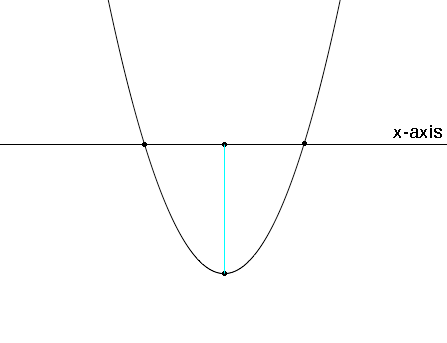SEARCH HOMEMath Central Quandaries & QueriesQuestion from rachel, a student: y=0.0008x^2-0.384x What is the minimum point of this equation?Rachel,

This is a quadratic equation so its graph is a parabola. First find x-intercepts, that is find the points on the graph that have y = 0. If y = 0 then

0.0008x2 - 0.384x = 0.

The left side of this expression has a common factor of x to it can be factored to yield

x (0.0008x- 0.384) = 0.

What are the points where the graph crosses the x-axis?By the symmetry of the parabola the x-coordinate of the minimum point is midway between the intercepts. What is the x-coordinate of the minimum point? What is the minimum value?

I hope this helps,
PennyMath Central is supported by the University of Regina and The Pacific Institute for the Mathematical Sciences.